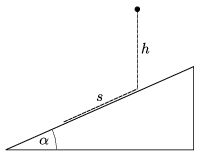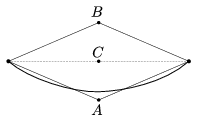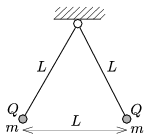Mathematical and Physical Journal
for High Schools
Issued by the MATFUND Foundation
 Already signed up? New to KöMaL?

# KöMaL Problems in Physics, April 2010

Show/hide problems of signs:## Problems with sign 'M'

Deadline expired on May 10, 2010.

M. 305. Measure how the refractive index of salt solution depends on its concentration.

(6 pont)

statistics## Problems with sign 'P'

Deadline expired on May 10, 2010.

P. 4244. A cat carefully approaches a plane mirror at a speed of 1 m/s. To her utmost surprise she realizes that her mirror image is moving away from her at a speed of 5 m/s, instead of approaching her, as she would expect. What can the explanation of this strange experience of the cat be?

(3 pont)

solution (in Hungarian), statistics

P. 4245. Why do we shorten our steps in winter, if the pavement is slippery?

(4 pont)

solution (in Hungarian), statistics

P. 4246. From an air gun a bullet is shot horizontally through the middle of a Plasticine sphere of 5 dkg, which is initially at rest on the edge of a table of height of 80 cm. The Plasticine sphere and the bullet of mass 0.5 g lands 8.2 m away from each other on the ground. In another experiment, in which the Plasticine sphere has three times bigger diameter, the bullet does not get through the sphere, but stops just at its surface. Determine the initial speed of the bullet, provided that it decelerates uniformly in the Plasticine in both cases.

(4 pont)

solution (in Hungarian), statistics

P. 4247. As the figure shows, a small object is dropped to a fixed, frictionless slope of angle of elevation=30o from a height h. The time of the fall is t1. After a totally inelastic collision the object slides down the slope. It covers the same distance s=h on the slope during the time t2. Determine the ratio of t1/t2.(4 pont)

solution (in Hungarian), statistics

P. 4248. In calm weather a rope, which is attached to a spherical balloon filled with Helium, hangs vertically and tight. In wind, however, in one case the rope makes an angle of 20o with the vertical, and in a second case an angle of 55o. Determine the ratio of the wind speeds in the two cases, provided that the air drag is proportional to the square of the speed of the wind.

(4 pont)

solution (in Hungarian), statistics

P. 4249. The two ends of a flexible rope of uniform density are fixed at the same height, as shown in the figure. Starting from the equilibrium position, we perform the following two experiments:

I. We pull down the midpoint of the rope till it reaches point A. (At this time the rope almost has a V-shape.)

II. From the equilibrium position we pull up the midpoint of the rope to the point B. (So now the shape of the rope is like an upside down V.)

The total work we did in the two processes is W. How much work is needed to lift the midpoint of the rope to the point C?(5 pont)

solution (in Hungarian), statistics

P. 4250. A cylindrical container is divided into two parts by a massless, frictionless, thermally insulating piston. In one part of the container there is 40 g Hydrogen, in the other part there is 20 g Neon, both in standard conditions.

a) Which gas, and to what temperature should be heated in order that the piston moves to the middle of the cylinder, provided that the temperature of the other gas does not change?

b) What is the final pressure of the gas which was heated?

c) How much heat was transferred to the gas?

(4 pont)

solution (in Hungarian), statistics

P. 4251. Two alike pendulums of length L are suspended at the same point. At the end of both pendulums there are small spheres of mass m. If both spheres are given the same Q charge, the threads move apart as it is shown in the figure.

a) Determine the mass m of the spheres.

b) In another experiment the sphere on the right is changed to another one of the same charge, but three times bigger mass. How should the charge of the left sphere be changed, so that in equilibrium the distance between the two spheres remains L? (The mass of the left sphere is not changed.)(5 pont)

solution (in Hungarian), statistics

P. 4252. A strongly short-sighted man wore on both eyes glasses of power -12 dioptres. The lenses were 2 cm from his eyes. In a cataract operation plastic lenses were implanted in his eyes, with which now he can see sharply the book held 25 cm away from his eyes. He is surprised by the fact, that he sees not only better than before, but he sees the book and the letters inside also bigger than before, with the glasses.

a) How much larger does the man see now the objects, which are 25 cm from his eyes, than before the operation he saw the objects, which were 25 cm away from his glasses?

b) Determine the power of the glasses (in dioptres) he needs to see sharply the Moon in the sky.

(5 pont)

solution (in Hungarian), statistics

P. 4253. How many electrons should be removed from a metal sphere of radius 1 cm, so that its electrostatic energy equals the energy loss due to mass defect? What would the electric potential of the sphere be in this case?

(4 pont)

solution (in Hungarian), statistics